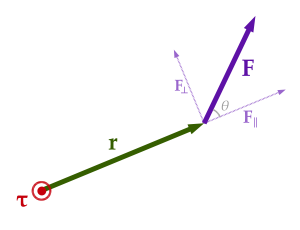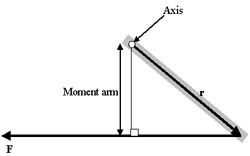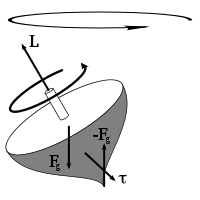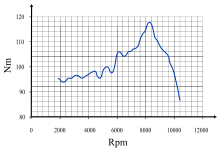# Torque

TorqueRelationship between force F, torque τ, linear momentum p, and angular momentum L in a system which has rotation constrained in one plane only (forces and moments due to gravity and friction not considered).

Torque, moment or moment of force (see the terminology below), is the tendency of a force to rotate an object about an axis, fulcrum, or pivot. Just as a force is a push or a pull, a torque can be thought of as a twist.

Loosely speaking, torque is a measure of the turning force on an object such as a bolt or a flywheel. For example, pushing or pulling the handle of a wrench connected to a nut or bolt produces a torque (turning force) that loosens or tightens the nut or bolt.

The symbol for torque is typically τ, the Greek letter tau. When it is called moment, it is commonly denoted M.

The magnitude of torque depends on three quantities: the force applied, the length of the lever arm connecting the axis to the point of force application, and the angle between the force vector and the lever arm. In symbols:$\boldsymbol \tau = \mathbf{r}\times \mathbf{F}\,\!$$\tau = rF\sin \theta\,\!$

where

τ is the torque vector and τ is the magnitude of the torque,
r is the displacement vector (a vector from the point from which torque is measured to the point where force is applied), and r is the length (or magnitude) of the lever arm vector,
F is the force vector, and F is the magnitude of the force,
× denotes the cross product,
θ is the angle between the force vector and the lever arm vector.

The length of the lever arm is particularly important; choosing this length appropriately lies behind the operation of levers, pulleys, gears, and most other simple machines involving a mechanical advantage.

The SI unit for torque is the newton metre (N·m). For more on the units of torque, see below.

## Terminology

This article follows US physics terminology by using the word torque. In the UK and in US mechanical engineering, this is called moment.

In US Mechanical Engineering, the term torque means 'the resultant moment of a Couple', and (unlike in US physics), the terms torque and moment are not interchangeable. Torque is defined mathematically as the rate of change of angular momentum of an object. The definition of torque states that one or both of the angular velocity or the moment of inertia of an object are changing. And moment is the general term used for the tendency of one or more applied forces to rotate an object about an axis, but not necessarily to change the angular momentum of the object (the concept which in physics is called torque).

For example, a rotational force applied to a shaft causing acceleration, such as a drill bit accelerating from rest, the resulting moment is called a torque. By contrast, a lateral force on a beam produces a moment (called a bending moment), but since the angular momentum of the beam is not changing, this bending moment is not called a torque. Similarly with any force couple on an object that has no change to its angular momentum, such moment is also not called a torque.

This article follows the US physics terminology by calling all moments by the term torque, whether or not they cause the angular momentum of an object to change.

## History

The concept of torque, also called moment or couple, originated with the studies of Archimedes on levers. The rotational analogues of force, mass, and acceleration are torque, moment of inertia and angular acceleration, respectively.

## Definition and relation to angular momentumA particle is located at position r relative to its axis of rotation. When a force F is applied to the particle, only the perpendicular component F produces a torque. This torque τ = r × F has magnitude τ = |r| |F| = |r| |F| sinθ and is directed outward from the page.

Torque is defined about a point not specifically about axis as mentioned in several books.

A force applied at a right angle to a lever multiplied by its distance from the lever's fulcrum (the length of the lever arm) is its torque. A force of three newtons applied two metres from the fulcrum, for example, exerts the same torque as a force of one newton applied six metres from the fulcrum. The direction of the torque can be determined by using the right hand grip rule: if the fingers of the right hand curl in the direction of rotation and the thumb points along the axis of rotation, then the thumb also points in the direction of the torque.

More generally, the torque on a particle (which has the position r in some reference frame) can be defined as the cross product:$\boldsymbol{\tau} = \mathbf{r} \times \mathbf{F},$

where r is the particle's position vector relative to the fulcrum, and F is the force acting on the particle. The magnitude τ of the torque is given by$\tau = rF\sin\theta,\!$

where r is the distance from the axis of rotation to the particle, F is the magnitude of the force applied, and θ is the angle between the position and force vectors. Alternatively,$\tau = rF_{\perp},$

where F is the amount of force directed perpendicularly to the position of the particle. Any force directed parallel to the particle's position vector does not produce a torque.

It follows from the properties of the cross product that the torque vector is perpendicular to both the position and force vectors. It points along the axis of rotation, and its direction is determined by the right-hand rule.

The unbalanced torque on a body along axis of rotation determines the rate of change of the body's angular momentum,$\boldsymbol{\tau} = \frac{\mathrm{d}\mathbf{L}}{\mathrm{d}t}$

where L is the angular momentum vector and t is time. If multiple torques are acting on the body, it is instead the net torque which determines the rate of change of the angular momentum:$\boldsymbol{\tau}_1 + \cdots + \boldsymbol{\tau}_n = \boldsymbol{\tau}_{\mathrm{net}} = \frac{\mathrm{d}\mathbf{L}}{\mathrm{d}t}.$

For rotation about a fixed axis,$\mathbf{L} = I\boldsymbol{\omega},$

where I is the moment of inertia and ω is the angular velocity. It follows that$\boldsymbol{\tau}_{\mathrm{net}} = \frac{\mathrm{d}\mathbf{L}}{\mathrm{d}t} = \frac{\mathrm{d}(I\boldsymbol{\omega})}{\mathrm{d}t} = I\frac{\mathrm{d}\boldsymbol{\omega}}{\mathrm{d}t} = I\boldsymbol{\alpha},$

where α is the angular acceleration of the body, measured in rad·s−2. This equation has the limitation that the torque equation is to be only written about instantaneous axis of rotation or center of mass for any type of motion - either motion is pure translation, pure rotation or mixed motion. I = Moment of inertia about point about which torque is written (either about instantaneous axis of rotation or center of mass only). If body is in translatory equilibrium then the torque equation is same about all points in the plane of motion.

### Proof of the equivalence of definitions

The definition of angular momentum for a single particle is:$\mathbf{L} = \mathbf{r} \times \mathbf{p}$

where "×" indicates the vector cross product, p is the particle's linear momentum, and r is the displacement vector from the origin (the origin is assumed to be a fixed location anywhere in space). The time-derivative of this is:$\frac{d\mathbf{L}}{dt} = \mathbf{r} \times \frac{d\mathbf{p}}{dt} + \frac{d\mathbf{r}}{dt} \times \mathbf{p}.$

This result can easily be proven by splitting the vectors into components and applying the product rule. Now using the definition of linear momentum p = mv (if mass is constant) and the definition of velocity$\frac{d\mathbf{r}}{dt} = \mathbf{v}$$\frac{d\mathbf{L}}{dt} = \mathbf{r} \times m \frac{d\mathbf{v}}{dt} + \mathbf{v} \times m\mathbf{v}.$

The cross product of any vector with itself is zero, so the second term vanishes. Hence with the definition of force F = ma (Newton's 2nd law),$\frac{d\mathbf{L}}{dt} = \mathbf{r} \times \mathbf{F}.$

Then by definition, torque τ = r × F.

If multiple forces are applied, Newton's second law instead reads Fnet = ma, and it follows that$\frac{d\mathbf{L}}{dt} = \mathbf{r} \times \mathbf{F}_{\mathrm{net}} = \boldsymbol{\tau}_{\mathrm{net}}.$

The proof relies on the assumption that mass is constant; this is valid only in non-relativistic systems in which no mass is being ejected.

## Units

Torque has dimensions of force times distance. Official SI literature suggests using the unit newton metre (N·m) or the unit joule per radian. The unit newton metre is properly denoted N·m or N m. This avoids ambiguity with mN, millinewtons.

The joule, which is the SI unit for energy or work, is dimensionally equivalent to a newton metre, but it is not used for torque. Energy and torque are entirely different concepts, so the practice of using different unit names for them helps avoid mistakes and misunderstandings. The dimensional equivalence of these units, of course, is not simply a coincidence: A torque of 1 N·m applied through a full revolution will require an energy of exactly 2π joules. Mathematically,$E= \tau \theta\$

where E is the energy, τ is magnitude of the torque, and θ is the angle moved (in radians). This equation motivates the alternate unit name joules per radian.

In British unit, "pound-force-feet" (lbf x ft), "foot-pounds-force", "inch-pounds-force", "ounce-force-inches" (oz x in) are used, and other non-SI units of torque includes "metre-kilograms-force". For all these units, the word "force" is often left out, for example abbreviating "pound-force-foot" to simply "pound-foot" (in this case, it would be implicit that the "pound" is pound-force and not pound-mass).

Sometimes one may see torque given units that don't dimensionally make sense. For example: g x cm . In these units, g should be understood as the force given by the weight of 1 gram at the surface of the earth. The surface of the earth is understood to have an average acceleration of gravity (approx. 9.80665 m/sec2).

## Special cases and other facts

### Moment arm formulaMoment arm diagram

A very useful special case, often given as the definition of torque in fields other than physics, is as follows:$|\tau| = (\textrm{moment\ arm}) (\textrm{force}).$

The construction of the "moment arm" is shown in the figure to the right, along with the vectors r and F mentioned above. The problem with this definition is that it does not give the direction of the torque but only the magnitude, and hence it is difficult to use in three-dimensional cases. If the force is perpendicular to the displacement vector r, the moment arm will be equal to the distance to the centre, and torque will be a maximum for the given force. The equation for the magnitude of a torque, arising from a perpendicular force:$|\tau| = (\textrm{distance\ to\ centre}) (\textrm{force}).$

For example, if a person places a force of 10 N on a spanner (wrench) which is 0.5 m long, the torque will be 5 N m, assuming that the person pulls the spanner by applying force perpendicular to the spanner.The torque caused by the two opposing forces Fg and −Fg causes a change in the angular momentum L in the direction of that torque. This causes the top to precess.

### Static equilibrium

For an object to be in static equilibrium, not only must the sum of the forces be zero, but also the sum of the torques (moments) about any point. For a two-dimensional situation with horizontal and vertical forces, the sum of the forces requirement is two equations: ΣH = 0 and ΣV = 0, and the torque a third equation: Στ = 0. That is, to solve statically determinate equilibrium problems in two-dimensions, we use three equations.

### Net force versus torque

When the net force on the system is zero, the torque measured from any point in space is the same. For example, the torque on a current-carrying loop in a uniform magnetic field is the same regardless of your point of reference. If the net force$\mathbf{F}$ is not zero, and$\mathbf{\tau}_1$ is the torque measured from$\mathbf{r}_1$, then the torque measured from$\mathbf{r}_2$ is$\mathbf{\tau}_2 = \mathbf{\tau}_1 + (\mathbf{r}_1 - \mathbf{r}_2) \times \mathbf{F}$

## Machine torqueTorque curve of a motorcycle ("BMW K 1200 R 2005"). The horizontal axis is the speed (in rpm) that the crankshaft is turning, and the vertical axis is the torque (in Newton metres) that the engine is capable of providing at that speed.

Torque is part of the basic specification of an engine: the power output of an engine is expressed as its torque multiplied by its rotational speed of the axis. Internal-combustion engines produce useful torque only over a limited range of rotational speeds (typically from around 1,000–6,000 rpm for a small car). The varying torque output over that range can be measured with a dynamometer, and shown as a torque curve. The peak of that torque curve occurs somewhat below the overall power peak. The torque peak cannot, by definition, appear at higher rpm than the power peak.

Understanding the relationship between torque, power and engine speed is vital in automotive engineering, concerned as it is with transmitting power from the engine through the drive train to the wheels. Power is a function of torque and engine speed. The gearing of the drive train must be chosen appropriately to make the most of the motor's torque characteristics. Power at the drive wheels is equal to engine power less mechanical losses regardless of any gearing between the engine and drive wheels.

Steam engines and electric motors tend to produce maximum torque close to zero rpm, with the torque diminishing as rotational speed rises (due to increasing friction and other constraints). Reciprocating steam engines can start heavy loads from zero RPM without a clutch.

## Relationship between torque, power and energy

If a force is allowed to act through a distance, it is doing mechanical work. Similarly, if torque is allowed to act through a rotational distance, it is doing work. Mathematically, for rotation about a fixed axis through the center of mass,$W = \int_{\theta_1}^{\theta_2} \tau\ \mathrm{d}\theta,$

where W is work, τ is torque, and θ1 and θ2 represent (respectively) the initial and final angular positions of the body. It follows from the work-energy theorem that W also represents the change in the rotational kinetic energy Krot of the body, given by$K_{\mathrm{rot}} = \tfrac{1}{2}I\omega^2,$

where I is the moment of inertia of the body and ω is its angular speed.

Power is the work per unit time, given by$P = \boldsymbol{\tau} \cdot \boldsymbol{\omega},$

where P is power, τ is torque, ω is the angular velocity, and · represents the scalar product.

Mathematically, the equation may be rearranged to compute torque for a given power output. Note that the power injected by the torque depends only on the instantaneous angular speed – not on whether the angular speed increases, decreases, or remains constant while the torque is being applied (this is equivalent to the linear case where the power injected by a force depends only on the instantaneous speed – not on the resulting acceleration, if any).

In practice, this relationship can be observed in power stations which are connected to a large electrical power grid. In such an arrangement, the generator's angular speed is fixed by the grid's frequency, and the power output of the plant is determined by the torque applied to the generator's axis of rotation.

Consistent units must be used. For metric SI units power is watts, torque is newton metres and angular speed is radians per second (not rpm and not revolutions per second).

Also, the unit newton metre is dimensionally equivalent to the joule, which is the unit of energy. However, in the case of torque, the unit is assigned to a vector, whereas for energy, it is assigned to a scalar.

### Conversion to other units

A conversion factor may be necessary when using different units of power, torque, or angular speed. For example, if rotational speed (revolutions per time) is used in place of angular speed (radians per time), we multiply by a factor of 2π radians per revolution.$\mbox{power} = \mbox{torque} \times 2 \pi \times \mbox{rotational speed}$$\mbox{power (W)} = \mbox{torque (N}\cdot\mbox{m)} \times 2 \pi \times \mbox{rotational speed (rps)}$

Dividing on the left by 60 seconds per minute and by 1000 watts per kilowatt gives us the following.$\mbox{power (kW)} = \frac{ \mbox{torque (N}\cdot\mbox{m)} \times 2 \pi \times \mbox{rotational speed (rpm)}} {60000}$

where rotational speed is in revolutions per minute (rpm).

Some people (e.g. American automotive engineers) use horsepower (imperial mechanical) for power, foot-pounds (lbf·ft) for torque and rpm for rotational speed. This results in the formula changing to:$\mbox{power (hp)} = \frac{ \mbox{torque(lbf}\cdot\mbox{ft)} \times 2 \pi \times \mbox{rotational speed (rpm)} }{33000}.$

The constant below in, ft·lbf/min, changes with the definition of the horsepower; for example, using metric horsepower, it becomes ~32,550.

Use of other units (e.g. BTU/h for power) would require a different custom conversion factor.

### Derivation

For a rotating object, the linear distance covered at the circumference in a radian of rotation is the product of the radius with the angular speed. That is: linear speed = radius × angular speed. By definition, linear distance=linear speed × time=radius × angular speed × time.

By the definition of torque: torque=force × radius. We can rearrange this to determine force=torque ÷ radius. These two values can be substituted into the definition of power:$\mbox{power} = \frac{\mbox{force} \times \mbox{linear distance}}{\mbox{time}}=\frac{\left(\frac{\mbox{torque}}{\displaystyle{r}}\right) \times (r \times \mbox{angular speed} \times t)} {t} = \mbox{torque} \times \mbox{angular speed}.$

The radius r and time t have dropped out of the equation. However angular speed must be in radians, by the assumed direct relationship between linear speed and angular speed at the beginning of the derivation. If the rotational speed is measured in revolutions per unit of time, the linear speed and distance are increased proportionately by 2π in the above derivation to give:$\mbox{power}=\mbox{torque} \times 2 \pi \times \mbox{rotational speed}. \,$

If torque is in lbf·ft and rotational speed in revolutions per minute, the above equation gives power in ft·lbf/min. The horsepower form of the equation is then derived by applying the conversion factor 33000 ft·lbf/min per horsepower:$\mbox{power} = \mbox{torque } \times\ 2 \pi\ \times \mbox{ rotational speed} \cdot \frac{\mbox{ft}\cdot\mbox{lbf}}{\mbox{min}} \times \frac{\mbox{horsepower}}{33000 \cdot \frac{\mbox{ft }\cdot\mbox{ lbf}}{\mbox{min}} } \approx \frac {\mbox{torque} \times \mbox{RPM}}{5252}$

because$5252.113122... = \frac {33000} {2 \pi}. \,$

## Principle of moments

The Principle of Moments, also known as Varignon's theorem (not to be confused with the geometrical theorem of the same name) states that the sum of torques due to several forces applied to a single point is equal to the torque due to the sum (resultant) of the forces. Mathematically, this follows from:$(\mathbf{r}\times\mathbf{F}_1) + (\mathbf{r}\times\mathbf{F}_2) + \cdots = \mathbf{r}\times(\mathbf{F}_1+\mathbf{F}_2 + \cdots).$

## Torque multiplier

A torque multiplier is a gear box, which works on the principle of epicyclic gearing. The given load at the input gets multiplied as per the multiplication factor and transmitted to the output, thereby achieving greater load with minimal effort.[citation needed]

Wikimedia Foundation. 2010.

### Look at other dictionaries:

• torque — [ tɔrk ] n. m. et f. • XIIIe ; lat. torques → torche 1 ♦ N. m. Archéol. Collier métallique rigide des Gaulois, puis des soldats romains. 2 ♦ N. f. (1419) Techn. Rouleau de fil de fer. ♢ (1690) Blas. Bourrelet d étoffe tortillée figurant le cimier …   Encyclopédie Universelle

• Torque — Torque, n. [L. torques a twisted neck chain, fr. torquere to twist.] 1. A collar or neck chain, usually twisted, especially as worn by ancient barbaric nations, as the Gauls, Germans, and Britons. [1913 Webster] 2. [L. torquere to twist.] (Mech.) …   The Collaborative International Dictionary of English

• torque — 1. (tor k ) s. f. 1°   Terme de blason. Bourrelet d étoffe tortillée, des deux principaux émaux de l écu, lequel se place quelquefois pour cimier sur le heaume qui couronne les armoiries. 2°   Botte de fil de laiton pliée en cercle. ÉTYMOLOGIE… …   Dictionnaire de la Langue Française d'Émile Littré

• torque — ► NOUN Mechanics ▪ a force that tends to cause rotation. ► VERB ▪ apply torque to. DERIVATIVES torquey adjective. ORIGIN from Latin torquere to twist …   English terms dictionary

• torque — [tôrk] n. [< L torques (infl. in senses 2 & 3 by torquere): see TORQUES] 1. a twisted metal collar or necklace worn by ancient Teutons, Gauls, Britons, etc. 2. Physics a measure of the tendency of a force to cause rotation, equal to the force… …   English World dictionary

• torque — [to:k US to:rk] n [U] [Date: 1800 1900; : Latin; Origin: torquere to twist ] technical the force or power that makes something turn around a central point, especially in an engine …   Dictionary of contemporary English

• torque — [ tɔrk ] noun uncount TECHNICAL the force that causes something to turn around a central point …   Usage of the words and phrases in modern English

• Torque — Le nom est porté dans le Nord Pas de Calais, mais on le rencontre aussi dans le domaine occitan (87, 07), où il faut sans doute le rattacher au verbe torcar (= frotter, nettoyer). Pour le nord de la France, on peut faire le même rapprochement,… …   Noms de famille

• torque — (n.) rotating force, 1884, from L. torquere to twist (see THWART (Cf. thwart)). The verb is attested from 1954. The word also is used (since 1834) by antiquarians and others as a term for the twisted metal necklace worn anciently by Gauls,… …   Etymology dictionary

• torque — s. m. 1. Bracelete ou colar, geralmente de ouro, usado por povos antigos. 2.  [Física] Tendência de uma força para rodar um objeto em torno de um eixo.   ‣ Etimologia: latim torques ou torquis, is, colar …   Dicionário da Língua Portuguesa